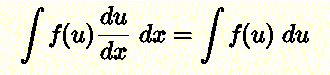# Rules of Integrals with Examples

A tutorial, with examples and detailed solutions, in using the rules of indefinite integrals in calculus is presented. A set of questions with solutions is also included.

In what follows, C is a constant of integration and can take any value.

## 1 - Integral of a power function: f(x) = x nExample: Evaluate the integral

x 5 dx

Solution:
x 5 dx = x5 + 1 / ( 5 + 1) + c = x 6 / 6 + c

## 2 - Integral of a function f multiplied by a constant k: k f(x)Example: Evaluate the integral

5 sinx dx

Solution:
According to the above rule
5 sin (x) dx = 5 sin(x) dx
sin(x) dx   is given by 2.1 in table of integral formulas , hence
5 sin(x) dx = - 5 cos x + C

## 3 - Integral of Sum of Functions.Example: Evaluate the integral

[x + e x] dx

Solution:
According to the above property
[x + e x ] dx = x dx + e x dx
x dx is given by 1.3 and e x dx by 4.1 in table of integral formulas , hence
[x + e x ] dx = x 2 / 2 + e x + c

## 4 - Integral of Difference of Functions.Example: Evaluate the integral

[2 - 1/x] dx

Solution:
According to the above property
[2 - 1/x] dx = 2 dx - (1/x) dx
2 dx is given by 1.2 and (1/x) dx by 1.4 in table of integral formulas , hence
[2 - 1/x] dx = 2x - ln |x| + c

## 5 - Integration by Substitution.Example: Evaluate the integral

(x 2 - 1) 20 2x dx

Solution:
Let u = x
2 - 1, du/dx = 2x and the given integral can be written as
(x 2 - 1) 20 2x dx
=
u 20 (du/dx) dx
=
u 20 du         according to above property
= u
21 / 21 + c = (x 2 - 1) 21 / 21 + c

## 6 - Integration by Parts.Example: Evaluate the integral

x cos x dx

Solution:
Let f(x) = x and g ' (x) = cos x which gives
f ' (x) = 1 and g(x) = sin x
From integration by parts formula above,
x cos x dx = x sin x - 1 sin x dx
= x sin x + cos x + c

## More Questions with Solutions

Use the table of integral formulas and the rules above to evaluate the following integrals. [Note that you may need to use more than one of the above rules for one integral].
1. (1 / 2) ln (x) dx
2. [sin (x) + x 5] dx
3. [sinh (x) - 3] dx
4. - x sin (x) dx

5. sin 10(x) cos(x) dx

## Solutions to the Above Questions

1.
This is the integral of ln (x) multiplied by 1 / 2 and we therefore use rule 2 above to obtain:
(1 / 2) ln (x) dx = (1 / 2) ln (x) dx
We now use formula 4.3 in the
table of integral formulas to evaluate ln (x) dx. Hence
(1 / 2) ln (x) dx = (1 / 2) ( (x ln (x)) - x ) + c

2.
Use rule 3 ( integral of a sum ) to obtain
[sin (x) + x 5 ] dx = sin (x) dx + x 5 dx
We use formula 2.1 in the
table of integral formulas to evaluate sin (x) dx and rule 1 above to evaluate x 5 dx. Hence
[sin (x) + x 5 ] dx = - cos (x) + x 6 / 6
3.
Use rule 4 (integral of a difference) to obtain
(sinh (x) - 3) dx = sinh (x) dx - 3 dx
We use formula 7.1 in the
table of integral formulas to evaluate sinh (x) dx and integral of the constant 3 to obtain
(sinh (x) - 3) dx = cosh (x) - 3 x + c
4.
The integrand is the product of two function x and sin (x) and we try to use integration by parts in rule 6 as follows:
Let f(x) = x , g'(x) = sin(x) and therefore g(x) = - cos(x)
Hence
- x sin (x) dx = - f(x) g'(x) dx = - ( f(x) g(x) - f'(x) g(x) dx)
Substitute f(x), f'(x), g(x) and g'(x) by x , 1, sin(x) and - cos(x) respectively to write the integral as
= - x (- cos(x)) +
1 (- cos(x)) dx
Use formula 2.2 in in the
table of integral formulas to evaluate cos(x) dx and simplify to obtain
= x cos (x) - sin(x) + c

5.
Let u = sin(x) and therefore du/dx = cos(x). Hence the given integral can be written as
sin 10 (x) cos dx = ( u 10 du/dx ) dx
Use rule 5 to write
=
u 10 du
which gives
= u
11 / 11 + c
Substitute u by sin(x) to obtain
= (1 / 11) (sin
11 (x) ) + c